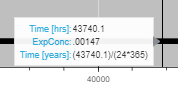# Hovertool help - Custom tooltip

Hi,

How do I display the time in years for the third hover information. I’ve tried several things but cant seem to figure it out. I want it to show years instead of hours so the user doesnt need to calculate it themselves. Where to I need to place the division of 24*365 in the tooltips formatter?
The code snippet is shown below and the result in the image below the code. Anyone know?

``````    tooltips_model = [
("Time [hrs]", "@x{(0.0)}"),
("ExpConc", "@ExpConc{(.00000)}"),
("Time [years]", "(@x{(0.0)})/(24*365)"),
]

``````Hi @Zana,

When I’ve had to do similar in the past, I’ve just added a new computed column to the data source instead. Then setting up the HoverTool is trivial.

Hi carolyn,

Hmm okej, so its not possible to change the format of a number by dividing it directly like I showed in the example?

You can also use a CustomJSHover to do a calculated value in a HoverTool. (Note that as of this posting, there’s a small error in the documentation for this that I will be fixing-- use the dict key format for `formatters` shown in the example below, and NOT what the reference guide says today.)

Here’s a basic example, where given a number of days, the tooltip returns hours:

``````from bokeh.plotting import figure, show
from bokeh.models import HoverTool, CustomJSHover
import numpy as np

x = [i for i in range(0, 10)]
days = np.random.randint(0, high=10, size=10)

p = figure()
p.line(x=x, y=days)

days_to_hours = CustomJSHover(code="""
var y = special_vars.data_y
return "" + (y*24)
""")